Welcome, parents!

Let your kids discover a fun math universe.

With Zapzapmath Home, your kids can enjoy hundreds of math games for Kindergarten to Grade 6, animated math lessons and tools. With just one Premium Membership, you get full access to all 11 Home apps below and future Zapzapmath Home apps.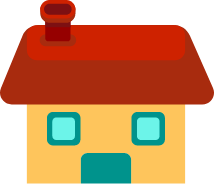Teachers?

Check out 'Zapzapmath School'
with Classroom reports!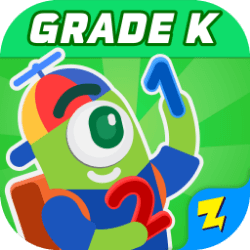In this app, kids learn to count, to use numbers to represent number of objects, addition and subtraction, measurable attributes, and shapes.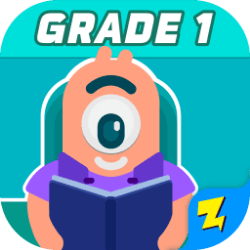In this app, kids learn to add and subtract, understand place value, measure and compare lengths, tell time, and understand shapes.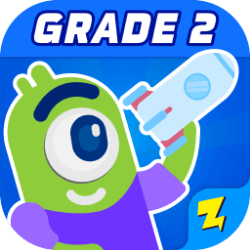In this app, kids work on multiplication, place values, understanding lengths, time and money problems, and reasoning with shapes.In this app, kids learn about multiplication and division, fractions, measurements, area, perimeter, and reasoning with shapes.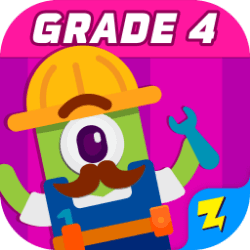In this app, kids learn about factor pairs, number patterns, rounding, equivalent fractions, decimals and classifying shapes.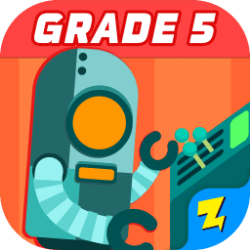In this app, kids learn about math expressions, converting measurements, understanding volume, coordinates, and classifying 2D figures.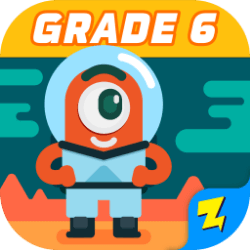In this app, kids learn about ratios, dividing fractions, common factors, equations, inequalities, and statistics.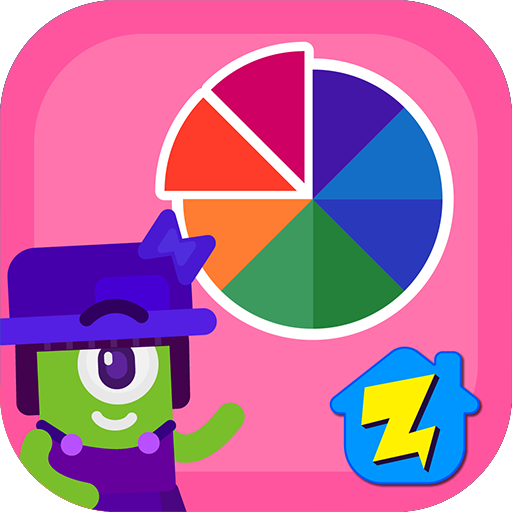Looking for a smart way to master the basics of fractions? Our free app teaches you core fraction concepts via videos.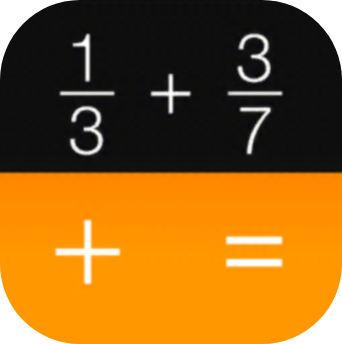Download Fraction Calculator + Decimals app here:Solve everyday fraction problems with functions like simplifying fractions, fractions-to-decimals and decimals-to-fractions.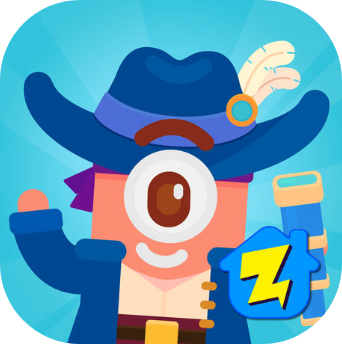Designed to change how kids think about learning math, here they will learn to multiply up to 12x12 and to compare values.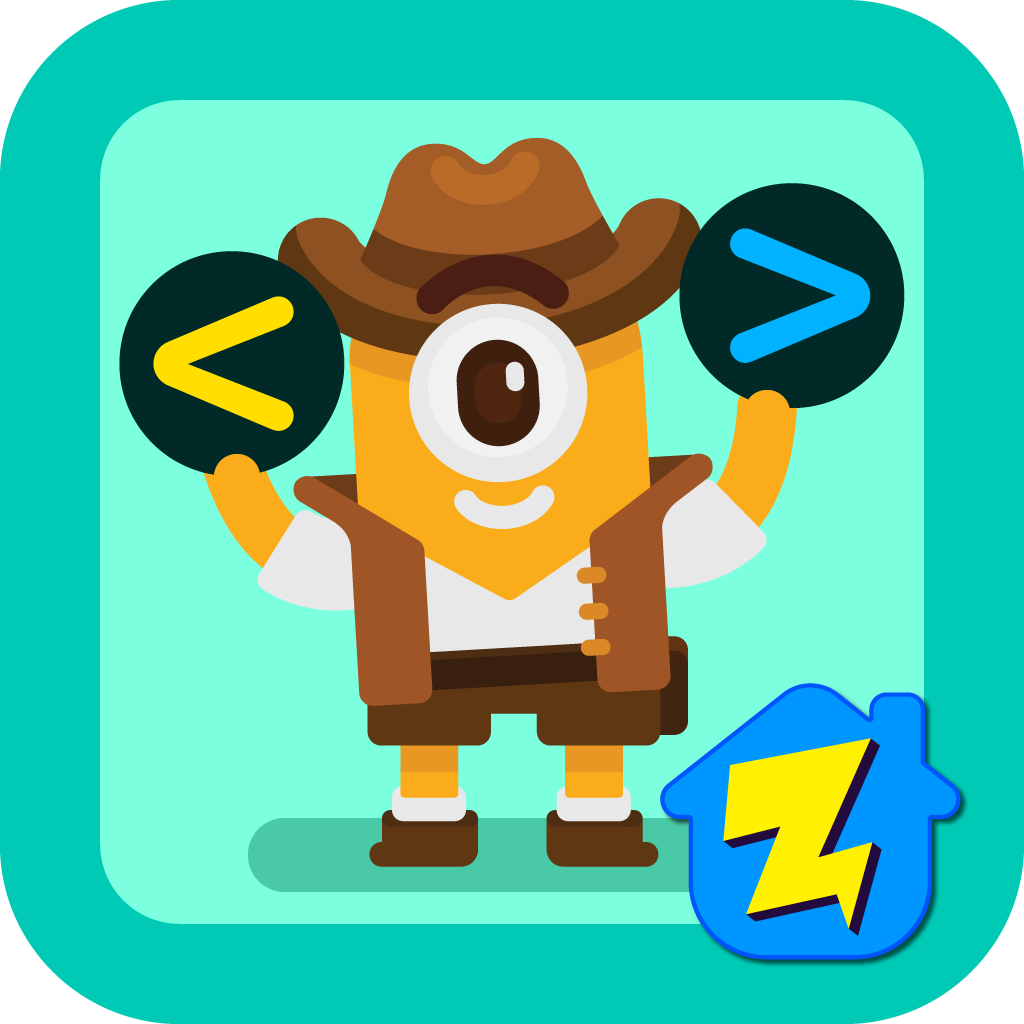In this research driven, fun and engaging app, kids improve their ability to recognize numbers and compare magnitude.

Upgrade to a Zapzapmath Home Membership to unlock a universe of math games across multiple apps.

Membership Benefits•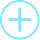All 100+ premium games unlocked

Access exclusive 100+ premium games that you can only get by being a member!

•Access all grades on Zapzapmath Home

••